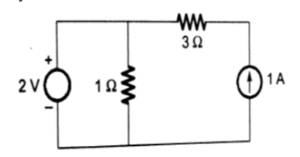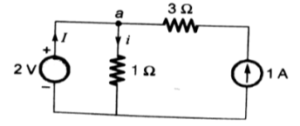Engineering Jobs   »   DFCCIL 2021, DFCCIL ELECTRICAL EXECUTIVE, DFCCIL...

# DFCCIL’21 EE: Daily Practices Quiz 23-Aug-2021

DFCCIL Recruitment 2021 –Dedicated Freight Corridor Corporation of India Limited (DFCCIL) Public Sector Undertaking under the administrative control of the Government of India (Ministry of Railways).DFCCIL has released the official notification for the recruitment of 1074 vacancies for the recruitment of Junior Executives, Executives, and Junior Managers through their official website, i.e. dfccil.com.

DFCCIL’21 EE: Daily Practices Quiz 23-Aug-2021

Each question carries 1 mark.
Negative marking: 1/4 mark
Total Questions: 06
Time: 06 min.

Q1. The relative permeability of a medium is equal to (with M=magnetization of medium and H=magnetic field strength)
(a) 1+M/H
(b) 1-M/H
(c) 1+√(M/H)
(d) 1-√(M/H)

Q2. For the circuit given in figure below the power delivered by the 2-volt source is given by(a) 4 W
(b) -4 W
(c) 2 W
(d) -2 W

Q3. If the torque/weight ratio of an instrument is low, then it can be concluded that
(a) The meter will have a uniform scale
(b) The meter will have the non-uniform scale
(c) The sensitivity of the meter will be high
(d) The sensitivity of the meter will be low

Q4. The Y_bus matrix of a 100-bus interconnected system is 80% sparse. Then, the number of transmission lines in the system must be
(a) 1500
(b) 1900
(c) 3000
(d) 950

Q5. An ideal transformer has an input voltage of 480V. The output current and voltage are 10A and 120V respectively. Determine the value of input current-
(a) 25 A
(b) 2.5 A
(c) 0.25 A
(d) 0.025 A

Q6. The secondary winding of which of the following transformers is always kept closed?
(a) Current transformer
(b) Voltage transformer
(c) Power transformer
(d) Step-down transformer

SOLUTIONS
S1. Ans.(a)
Sol. Magnetic susceptibility(X)=M/H=µ_r-1
Where; µ_r=relative permeability
∴µ_r=1+X=1+M/H

S2. Ans.(c)
Sol.Here, i=2/1=2 A
On applying KCL at node a: I=i-1=2-1=1 A
i.e., the current delivered by the voltage source=1 A
∴power delivered by the voltage source=2×1=2 W

S3. Ans.(d)
Sol. The low torque/weight ratio signifies heavy weight of the moving system due to which the instrument incurs frictional losses hence the sensitivity will be low.

S4. Ans.(d)
Sol. Number of buses, N=100
Sparsity, s=0.8
Then, the number of transmission lines is given as: N_T=(N^2 (1-s)-N)/2=(100^2 (1-0.8)-100)/2
⇒N_T=(100×19)/2=950

S5. Ans.(b)
Sol. V₁I₁ = V₂I₂
⇒ I₁ = (V₂I₂)/(V₁)
=(120×10)/480=2.5 A

S6. Ans.(a)
Sol. Because if CT secondary is not shorted then it can develop a very high voltage across secondary which may damage transformer insulation.

Sharing is caring!

Thank You, Your details have been submitted we will get back to you.